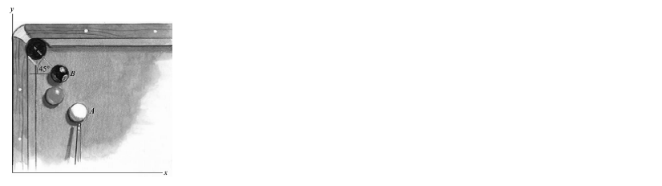## what are the velocity vectors of the two balls just after the impact?

The cue gives the cue ball A a velocity parallel to the y-axis. The cue ball hits the eight ball B and knocks it straight into the corner pocket. If the magnitude of the velocity of the cue ball just before the impact is 2 m/s and the coecient of restitution is e = 0:9, what are the velocity vectors of the two balls just after the impact? (The balls are of equal mass.)Solved